# Magneto-Hemodynamic of Blood Flow Having Impact of Radiative Flux Due to Infrared Magnetic Hyperthermia: Spectral Relaxation Approach

Magneto-Hemodynamic of Blood Flow Having Impact of Radiative Flux Due to Infrared Magnetic Hyperthermia: Spectral Relaxation Approach

Ebenezer O. Ige Funmilayo H. Oyelamisup* Joshua Olutayo-Irheren Joseph T. Okunlola

Department of Biomedical Engineering, Afe Babalola University, Ado-Ekiti 360231, Nigeria

Department of Mechanical and Mechatronics Engineering, Afe Babalola University, Ado-Ekiti 360231, Nigeria

Department of Mathematical and Physical Sciences, Afe Babalola University, Ado-Ekiti 360231, Nigeria

Corresponding Author Email:
Page:
773-779
|
DOI:
https://doi.org/10.18280/ijdne.170516
1 June 2022
|
Accepted:
15 August 2022
|
Published:
31 October 2022
| Citation

OPEN ACCESS

Abstract:

Hyperthermia therapy is an adjuvant procedure during which perfused body tissues is subjected to elevated range of temperature in bid to achieve improved drug potency and efficacy of cancer treatment. While a selected class of hyperthermia techniques is shouldered on the thermal radiations derived from single-sourced electro-radiation measures, there are deliberations on conjugating dual radiation field sources in an attempt to improve the delivery of therapy procedure. This paper numerically explores the thermal effectiveness of combined infrared hyperemia having nanoparticle recirculation in the vicinity of imposed magnetic field on subcutaneous strata of a model lesion as ablation scheme. An elaborate Spectral relaxation method (SRM) was formulated to handle equation of coupled momentum and thermal equilibrium in the blood-perfused tissue domain of a spongy fibrous tissue. Thermal diffusion regimes in the presence of external magnetic field imposition were described leveraging on the renowned Roseland diffusion approximation to delineate the impact of radiative flux within the computational domain. The contribution of tissue sponginess was examined using mechanics of pore-scale porosity over a selected of clinical informed scenarios. Our observations showed for a substantial depth of spongy lesion, magnetic field architecture constitute the control regimes of hemodynamics in the blood-tissue interface while facilitating thermal transport across the depth of the model lesion. This parameter-indicator could be utilized to control the dispensing of hyperthermia treatment in intravenous perfused tissue.

Keywords:

spectra relaxation scheme, thermal equilibrium, Roseland diffusion approximation

1. Introduction

The science of blood fluid dynamics in the presence of static high magnetic fields is known as magneto-hemodynamics. The importance of this study stems from the fact that hemodynamic dysfunction causes the majority of cardiovascular ailments such as stenosis and abnormalities, leading to cancer. Hyperthermia is a treatment that involves exposing bodily tissue to high temperatures in order to harm and kill cancer cells or make them simpler to kill with radiation therapy or chemotherapy. Hyperthermia treatment can take several forms, including putting the full body or a portion of it in a heated chamber or machine, as pictured.

According to the World Health Organization, cancer-related death is projected to surge by 15% across the globe for the period 2010-2020. According to the Surveillance, Epidemiology, and End Results (SEER) initiative of the National Cancer Institute (NCI), cancer incidence affects roughly 9% of the US population. In Europe, Ferlay et al.  reported approximate death tool of 1.9 million and nearly 4 million new cases for the 2018. While nations in the developed world are achieving substantial progress in cancer management, Africa is currently experiencing rapid surge in cancer incidence. For instance, experts in the field of oncology in Africa reported that Nigeria has the highest mortality in age-standardized study . However, the improvement on thermal and non-thermal therapies in diagnosis and management of cases of tumor-related illnesses is suggested to hold the potential to douse the mortality burden associated with patients inhibited with cancerous infections. Present hope in combating cancer plague is triggered by capabilities of several strategies such as hyperthermic perfusion, conductive hyperthermia, electromagnetic and ultrasonic hyperthermia and electrothermic procedures.

Hyperthermia (HT) has been an important strategy to treat cancer-related illness over the years. Innovations around applications of thermal biology for tumor in several anatomical sites of the body have been reported. High temperature inducement of malignant region is described as an adjunctive procedure where elevated temperature field exposure on portions of tumor growth is administered to provide curative effect on the tissue . To improve the potency of clinical interventions with far-reaching therapeutics effects such as chemotherapy and radiotherapy , used finite element method for the computation of magneto-hemodynamic flow and heat transfer in a bifurcated artery with saccular aneurysm using the Carreau-Yasuda biorheological model. Ige et al.  examined nanoparticle-embedded blood flow control with magnetic field influence using spectra homotopy analysis. Using implantable anticancer drug delivery with thermal ablation, Al-Zu’bi and Mohan  studied Modelling of combination therapy in solid tumor. Shorif et al.  investigated magnetic nanoparticles mediated cancer hyperthermia while the Study of heat and mass transfer to magnetohydrodynamic (MHD) pulsatile couple stress fluid between two parallel porous plates was considered by Oyelami et al. . In the treatment planning of radiofrequency hyperthermia therapy in clinics  studied the role of simulations. Subject to a transient laser irradiation, Zhang et al.  examined modelling and simulation on heat transfer in blood vessels. In order to improve the accumulation and penetration of magnetic nanocarriers into solid tumors, Liu et al.  used oppositely polarized external magnets in his analysis. Magneto-Hemodynamics Mixed Convection with radiative heat transfer in a stenosed artery was investigated by Ngufor and Makinde . Nguyen et al.  reported a non-invasive HT strategy for mammalian cancer using microwave HT in a three-dimensional antenna-beam approach to describe electromagnetic wave penetration in breast cancer model. Nguyen et al.  experimentally showed that 65-W microwave HT penetration for breast cancer treatment by focusing 3D-antenna in the vicinity of tumor embedded in glands at elevated temperature 42℃.

During hyperthermia-type treatment in cancer issues, perfusion of blood across the vasculature of the diseased region is regarded because of its impact for circulation and removal of thermal energy in the vicinity of neighboring tissue. Numerical procedures have the capacity to predict and provide guidance to clinicians during administration of hyperthermia therapy in cancer patients.

In this study, investigation on the impact of magnetic field mediated radiative flux on infrared-type hyperthermia on a model spongy tissue under perfusion is presented and implemented by Chebyshev and pseudo-Spectral relaxation scheme. The elucidation of transport of biogenic fluid across the microvasculature of the perfused lesion is captured in our investigation. For the first time, we report field-based augmentation infrared-mediated hyperthermia with externally imposed magnetic field in infrared-mediated. While the perfusion in a near-native vascular spongy tissue is considered with predicated degree of porosity to analyzed the impact of controlled infrared over selected permeability of the substrate lesion.

2. Formulation of the Research Problem

Consider hyperthermia therapy having the existence of magnetic field. The blood tissue is presumed to be at a uniform temperature of 37℃ and the heat source is considered in the study so as to serve as an external device to increase the temperature. This mechanism of heat transfer combines convection, perfusion of blood, thermal conduction and metabolic heat generation. The previous work  neglected the blood tissue momentum and the viscous dissipation. Hence, the present study elucidates the momentum and thermal equilibrium that takes place between blood and tissue. This study assumes that the hotness of blood throughout the tissue is comparable to the hotness of the local tissue. In order to maximize the design and operation of heat exchangers, engineers and researchers need accurate heat transfer correlations . Thus, the one-dimensional momentum and energy equations becomes:

$\frac{\partial \bar{u}}{\partial \bar{t}}=v \frac{\partial^2 \bar{u}}{\partial \bar{y}^2}-\frac{v}{K} \bar{u}-\frac{\sigma B_o^2}{\rho} \bar{u}$              (1)

$\rho_b C_{p b} \frac{\partial \bar{T}}{\partial \bar{t}}=K_b \frac{\partial^2 \bar{T}}{\partial \bar{y}^2} \omega_b \rho C_b\left(T_b-T\right)+Q_m\left(\bar{T}-T_o\right)+\frac{\partial q_r}{\partial y}+\mu\left(\frac{\partial \bar{u}}{\partial \bar{y}}\right)$           (2)

along with the boundary conditions:

$\bar{u}(\bar{y}, 0)=37^{\circ} \mathrm{C}, \bar{u}(0, \bar{t})=37^{\circ} \mathrm{C}, \bar{u}(a, \bar{t})=45^{\circ} \mathrm{C}$           (3)

$\bar{T}(\bar{y}, 0)=37^{\circ} \mathrm{C}, \bar{T}(0, t)=37^{\circ} \mathrm{C}, \bar{T}(a, \bar{t})=45^{\circ} \mathrm{C}$                  (4)

The Rosseland diffusion approximation as used in  is employed on the radiative heat flux and defined as:

$q_r=\frac{-4 \sigma_S}{3 K_e} \frac{\partial T^4}{\partial \bar{y}}$               (5)

where, the Stefan-Boltzman constant is σ and Ke signifies mean absorption coefficient. When the Taylor series is used to expand T4 and higher orders are ignored, it is presumed that temperature modifications within the blood motion are adequately minimal that T4 may be represented as a linear function.

We obtain:

$T^4 \cong 4 T_b^3 \bar{T}-3 T_h^4$                  (6)

Substituting Eq. (6) into (2) to obtain:

$\frac{\partial q_r}{\partial \bar{y}}=\frac{-16 \sigma_s T_b^3}{3 K_e} \frac{\partial^2 \bar{T}}{\partial \bar{y}^2}$                       (7)

The non-dimensional functions enumerated below are established.

$u=\frac{\bar{u}}{v}, y=\frac{\bar{y}}{v}, t=\frac{\bar{t}}{v^2}, \theta=\frac{\bar{T}-T_0}{T_b-T_0}$          (8)

Using Eq. (8), Eq. (1) and (2) in their dimensionless form becomes:

$\frac{\partial u}{\partial t}=\frac{\partial^2 u}{\partial y^2}-\frac{1}{K \rho} u-m u$                  (9)

$\frac{\partial \theta}{\partial t}=\alpha\left(1+\frac{4}{3} R\right) \frac{\partial^2 \theta}{\partial y^2}+(r+\beta)-(r-\lambda) \theta+E_c\left(\frac{\partial u}{\partial v}\right)^2$                          (10)

where, $\alpha=\frac{K_b}{\rho_b C_{p b}}, \beta=\frac{\varepsilon a^2}{K \rho_b C_{p b}\left(T_b-T_0\right)}, r=a^2 \omega_b, \lambda=\frac{Q m a^2}{\rho_b C_{p b}}$.

Each of the parameters are defined as follows: $\bar{u}$ means velocity component in $\bar{y}$ direction, $\bar{t}$ means time, ν means fluid viscosity, K means permeability coefficient, σ means electrical conductivity, Bo is the magnetic field, ρ is the density, Cpb means specific heat at constant pressure, Kb is the thermal conductivity, $\bar{T}$ is the fluid temperature, Tb is the ambient temperature, Qm is the heat generation coefficient, qr is the radiative heat flux, μ is the kinematic velocity.

3. Method of Approach (Spectral Relaxation Method)

The SRM is utilized to provide answer to the transformed non - dimensional Eqns. (9) and (10). This is an iterative technique for linearizing and decoupling sets of nonlinear differential equations by employing Gauss-Siedel relaxation procedure. The linear system of equations are then discretized using , a Chebyshev pseudo-spectral approach. The linear functions are calculated at the recent iteration step (r+1), but the non-linear functions are presumed to exist at the previous step (r).

The fact that this method (SRM) can solve both ordinary and partial differential equations makes it unique. Apply the SRM to the modified momentum and energy Eqns. (9) and (10) to get Eqns. (11) and (12) as shown below:

$\frac{\partial u_{r+1}}{\partial t}=\frac{\partial^2 u_{r+1}}{\partial y^2}-\frac{1}{K_p} u_{r+1}-m u_{r+1}$          (11)

$\frac{\partial \theta_{r+1}}{\partial t}=\alpha\left(1+\frac{4}{3} R\right) \frac{\partial^2 \theta_{r+1}}{\partial y^2}+(r+\beta)-(r-\lambda) \theta_{r+1}+E_c\left(\frac{\partial u}{\partial y}\right)^2$              (12)

Subject to:

$u_{r+1}(y, 0)=37^{\circ} \mathrm{C}, u_{r+1}(0, t)=37^{\circ} \mathrm{C}$               (13)

$\theta_{r+1}(y, 0)=37^{\circ} \mathrm{C}, \theta_{x+1}(0, t)=37^{\circ} \mathrm{C}$               (14)

Setting:

$a_{0, r}=\frac{1}{K_p}, a_{1, r}=-m, b_{0, r}=\alpha\left(1+\frac{4}{3} R\right), b_{1, r}=-(r-\lambda)$

$b_{2, r}=(\beta+r), b_{3, r}=E_c\left(\frac{\partial u}{\partial y}\right)^2$

Substituting the above coefficient parameters into (11) and (12) to obtain:

$\frac{\partial u_{r+1}}{\partial t}=\frac{\partial^2 u_{r+1}}{\partial y^2}+a_{0, r} u_{r+1}+a_{1, r} u_{r+1}$                (15)

$\frac{\partial \theta_{r+1}}{\partial t}=b_{0, r} \frac{\partial^2 \theta_{r+1}}{\partial y^2}+b_{1, r} \theta_{r+1}+b_{2, r}+b_{3, r}$               (16)

Subject to (13) and (14).

We proceed to define the Gauss-Lobatto points as given by Idowu and Falodun  as:

$\Sigma_j=\cos \frac{\pi j}{N}, j=0,1,2, \ldots, N ; 1 \leq \xi \leq-1$                    (17)

The realm of the physical neighborhood is converted into [-1, 1] from [0, 1], wherein N stand for number of collocation points.

In reference to the boundary conditions, an initial guess is chosen as:

$u_0(y, t)=\theta_0(y, t)=37^{\circ} \mathrm{C}+e^{-\eta}-1$                 (18)

As a result, starting with the initial approximation defined in Eq. (8), the dimensionless Eqns. (9) and (10) were solved iteratively for the unknown functions (18). Whenever r=0, 1, 2, the iterative schemes (15) and (16) are solved iteratively for ur+1(y,t) and θr+1(y,t) respectively. The Chebyshev spectral collocation method will be used to discretize the equations in the y-path, as well as the implicit difference approach for centering mid-point within tn+1 and tn.

The midpoint is conveyed as:

$t^{n+\frac{1}{2}}=\frac{t^{n+1}+t^n}{2}$               (19)

Thus, using the centering point about $t^{n+\frac{1}{2}}$ to the unidentified functions, say u(y,t) and θ(y,t) with its associated derivative as noted by Alao et al.  and defined in this study as:

$u\left(y_j, t^{n+\frac{1}{2}}\right)=u_j^{n+\frac{1}{2}}=\frac{u_j^{n+1}}{2},\left(\frac{\partial u}{\partial t}\right)^{n+\frac{1}{2}}=\frac{u_j^{n+1}-u_j^n}{\Delta t}$                 (20)

$\theta\left(y_j, t^{n+\frac{1}{2}}\right)=\theta_j^{n+\frac{1}{2}}=\frac{\theta_j^{n+1}}{2},\left(\frac{\partial \theta}{\partial t}\right)^{n+\frac{1}{2}}=\frac{\theta_j^{n+1}-\theta_j^n}{\Delta t}$                     (21)

We proceed to apply the concept of spectral collocation method to estimate derivatives of unidentified variables given in (22) and (23) using differential matrix D:

$\frac{d^r u}{d v^r}=\sum_{K=0}^N D_{i K}^r u\left(\varepsilon_K\right)=D^r u, i=0,1, \ldots, N$                (22)

$\frac{d^r \theta}{d y^r}=\sum_{K=0}^N D_{i K}^r \theta\left(\varepsilon_K\right)=D^r \theta, i=0,1, \ldots, N$             (23)

Applying Eqns. (22) and (23) on (15) and (16) before applying the finite difference scheme to obtain:

$\frac{d u_{r+1}}{d t}=u_{r+1} D^2+a_{0, r} u_{r+1}+a_{1, r} u_{r+1}$                        (24)

$\frac{d \theta_{r+1}}{d t}=b_{0, r} D^2 \theta_{r+1}+b_{1, r} \theta_{r+1}+b_{2, r}+b_{3, r}$                       (25)

Subject to:

$u_{r+1}\left(x_0, t\right)=37^{\circ} \mathrm{C}, u_{r+1}\left(x N_r, t\right)=37^{\circ} \mathrm{C}$                    (26)

$\theta_{r+1}\left(x_0, t\right)=37^{\circ} \mathrm{C}, \theta_{r+1}\left(x N_x, t\right)=37^{\circ} \mathrm{C}$              (27)

Simplifying Eqns. (24) and (24) to obtain:

$\frac{d u_{r+1}}{d t}=\left(D^2+a_{0, r}+a_{1, r}\right) u_{r+1}$         (28)

$\frac{d \theta_{r+1}}{d t}=\left(b_{0, r} D^2+b_{1, r}\right) \theta_{r+1}+b_{2, r}+b_{3, r}$                 (29)

We proceed to apply the finite difference scheme to obtain:

$\left(\frac{u_{r+1}^{n+1}-u_{r+1}^n}{\Delta t}\right)=\left(D^2+a_{0, r}+a_{1, r}\right)\left(\frac{u_{r+1}^{n+1}+u_{r+1}^n}{2}\right)$                (30)

$\left(\frac{\theta_{r+1}^{n+1}-\theta_{r+1}^n}{\Delta t}\right)=\left(b_{o, r} D^2+b_{1, r}\right)\left(\frac{\theta_{r+1}^{n+1}+\theta_{r+1}^n}{2}\right)+b_{2, r}+b_{3, r}$               (31)

Simplifying further to obtain:

$\left(\frac{1}{\Delta t}\right) u_{r+1}^{n+1}-\left(\frac{1}{\Delta t}\right) u_{r+1}^n=\left(\frac{D^2+a_{o, r}+a_{1, r}}{2}\right) u_{r+1}^{n+1}+\left(\frac{D^2+a_{o, r}+a_{1, r}}{2}\right) u_{r+1}^n$               (32)

$\left(\frac{1}{\Delta t}\right) \theta_{r+1}^{n+1}-\left(\frac{1}{\Delta t}\right) \theta_{r+1}^n=\left(\frac{b_{o, r} D^2+b_{1, r}}{2}\right) \theta_{r+1}^{n+1}+\left(\frac{b_{o, r} D^2+b_{1, r}}{2}\right) \theta_{r+1}^n+b_{2, r}+b_{3, r}$            (33)

Upon further simplification,

$\left(\frac{1}{\Delta t}\right) u_{r+1}^{n+1}-\left(\frac{D^2+a_{o, r}+a_{1, r}}{2}\right) u_{r+1}^{n+1}=\left(\frac{1}{\Delta t}\right) u_{r+1}^n+\left(\frac{D^2+a_{o, r}+a_{1, r}}{2}\right) u_{r+1}^n$              (34)

$\left(\frac{1}{\Delta t}\right) \theta_{r+1}^{n+1}-\left(\frac{b_{o, r} D^2+b_{1, r}}{2}\right) \theta_{r+1}^{n+1}=\left(\frac{1}{\Delta t}\right) \theta_{r+1}^n+\left(\frac{b_{o, r} D^2+b_{1, r}}{2}\right) \theta_{r+1}^n+b_{2, r}+b_{3, r}$                 (35)

Simplifying further to obtain:

$\left[\left(\frac{1}{\Delta t}\right)-\left(\frac{D^2+a_{o, r}+a_{1, r}}{2}\right)\right] u_{r+1}^{n+1}=\left[\left(\frac{1}{\Delta t}\right)+\right.\left.\left(\frac{D^2+a_{o, r}+a_{1, r}}{2}\right)\right] u_{r+1}^n+K_1$               (36)

$\left[\left(\frac{1}{\Delta t}\right)-\left(\frac{b_{o, r} D^2+b_{1, r}}{2}\right)\right] \theta_{r+1}^{n+1}=\left[\left(\frac{1}{\Delta t}\right)+\right.\left.\left(\frac{b_{o, r} D^2+b_{1, r}}{2}\right)\right] \theta_{r+1}^n+K_2$                     (37)

Hence, the iterative scheme becomes:

$A_1 u_{r+1}^{n+1}=B_1 u_{r+1}^n+K_1$                 (38)

$A_2 \theta_{r+1}^{n+1}=B_2 \theta_{r+1}^n+K_2$           (39)

where,

$A_1=\left[\left(\frac{1}{\Delta t}\right)-\left(\frac{D^2+a_{o, r}+a_{1, r}}{2}\right)\right], B_1=\left[\left(\frac{1}{\Delta t}\right)+\right.\left.\left(\frac{D^2+a_{o, r}+a_{1, r}}{2}\right)\right], K_1=0$

$A_2=\left[\left(\frac{1}{\Delta t}\right)-\left(\frac{b_{o, r} D^2+b_{1, r}}{2}\right)\right], B_2=\left[\left(\frac{1}{\Delta t}\right)+\right.\left.\left(\frac{b_{o, r} D^2+b_{1, r}}{2}\right)\right], K_2=b_{2, r}+b_{3, r}$

4. Results and Discussion

Utilizing the SRM, this study investigated hyperthermia therapy with magnetic field strength. In order to investigate the physics of the model, the effects of relevant flow parameters were visually illustrated. The numerical method employed in this work is an iterative method that decouples the system's equations using the Gauss Seidel approach.

The variation of heat source parameters on the temperature field is portrayed in Figure 1. Membrane pumps generate heat in the tissue, which is useful for active transport as well as the energy required for chemical reactions. The key region where heat source in tissue plays a big role is convective heat dissemination within the blood and the forearm tissue. In a heat steady state of prejudiced immersion in water at 38°C, the blood acts as a heat sink, transferring heat aside from the limb; the amount of heat earned from the vicinity is negligible. As a result, as the heat source parameter is increased, the fluid temperature drops.

Figure 2 illustrates that as the blood perfusion parameter rises, the temperature distribution rises as well. It thus implies that as blood perfusion increases, fluid velocity reaches its maximum. This means that at r=0.3, the biological tissues rise and become linear, whereas at r=0.1, the biological tissues decrease and become linear. At r=0.1, 0.2, and 0.3, Figure 3 shows that increasing the blood rate of perfusion induces an elevation in the fluid temperature of natural tissue.

A pressure cuff is used to control perfusion pressure by occluding the iliac artery. Direct measurement of blood flow temperature and velocity profile is the most common method for determining blood flow. It is crucial to note that when the temperature is high, the blood viscosity decreases, resulting in an increase in the temperature plot. Figure 4 depicts the influence of a magnetic field on temperature plot. The temperature profile appears to be unaffected by the rise in magnetic field. Figure 5 portrayed the reaction of the magnetic field on the velocity plot. The velocity profile is shown to rise as the magnetic parameter is increased. This simply indicates that at the highest magnetic parameter, the Lorentz force, which causes the fluid velocity to drop, is zero. This means that the magnetic field parameter's presence and application have a significant impact on the velocity plot. The reaction of increasing the permeability term has no influence on the temperature field in Figure 6. The reaction of the porosity term on the velocity plot is seen in Figure 7. The velocity profile accelerates as the porosity parameter is increased. This is due to the fact that the porous tissue that causes blood cell migration is accelerating. The dependence of the radiation on the velocity plot is shown in Figure 8. The velocity plot is found to be unaffected by raising the radiation term. The effect of radiation on the temperature plot is seen in Figure 9. A higher radiation spurs the temperature plot to rise. At higher temperatures, the radiation parameter becomes quite important. Figure 10 portrayed the influence of the heat source on velocity. The velocity plot is observed to grow as the heat source parameter is increased. The influence of heat conductivity on the velocity profile is characterized in Figure 11. It has been detected that as heat conductivity rises, the velocity profile rises as well.

## 1.jpg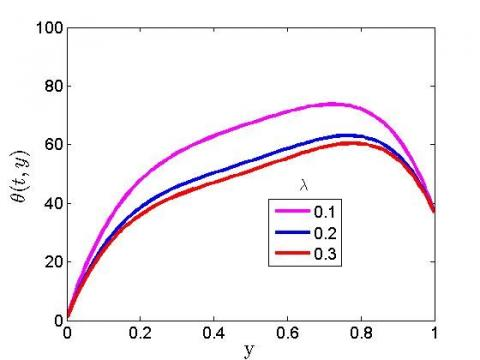Figure 1. The significance of heat source on temperature plot

## 2.jpgFigure 2. The significance of blood perfusion parameter on velocity plot

## 3.jpgFigure 3. The significance of blood perfusion on temperature plot

## 4.jpg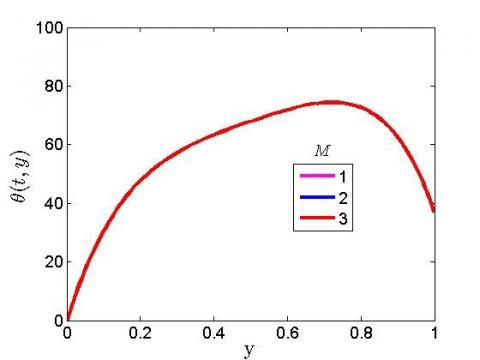Figure 4. The significance of magnetic field on temperature plot

## 5.jpg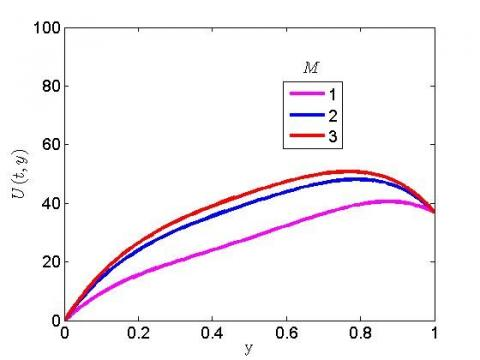Figure 5. The significance of magnetic field on velocity plot

## 6.jpg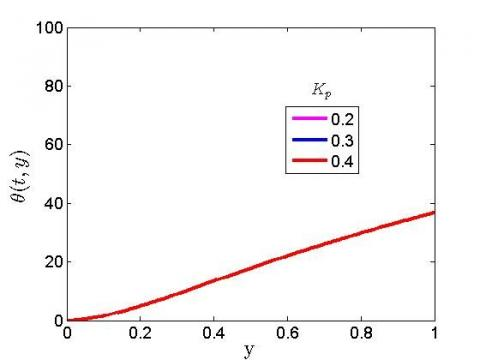Figure 6. The significance of the porosity parameter on temperature plot

## 7.jpg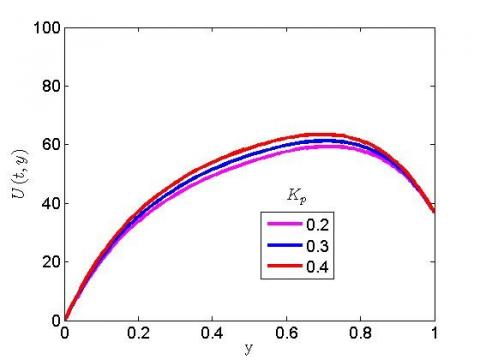Figure 7. The significance of porosity parameter on velocity plot

## 8.jpg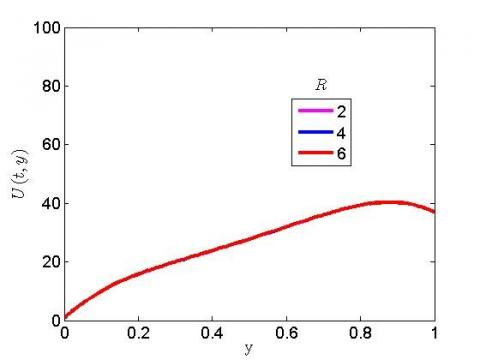Figure 8. The significance of radiation parameter on velocity plot

## 9.jpgFigure 9. The significance of radiation parameter on temperature plot

## 10.jpgFigure 10. The significance of heat source on velocity plot

## 11.jpgFigure 11. The significance of thermal conductivity on velocity plot

5. Conclusions

Investigation on the impact of radiative flux due to magnetic and infrared hyperthermia has been investigated under the influence of porosity term numerically. We considered the blood tissue at a temperature of 37℃ while heat source is studied to serve as an external device to intensify the temperature.

After critical examination of the problem, the following are the key findings:

(1) An incremental value of the heat source reduces the temperature;

(2) The blood viscosity reduces when there is high temperature, this signifies elevation in the temperature plot;

(3) A higher magnetic parameter was found to degenerate the velocity plot but has no significance on the temperature profile;

(4) The permeable tissue spurs the movement of the blood cell to accelerate;

(5) A higher thermal radiation was found to hike the fluid temperature profile but has no effect on the velocity profile.

Acknowledgment

The authors are grateful to management of Afe Babalola University Ado-Ekiti Nigeria for providing facilities to conduct this research. The contribution of Dr. Bidemi Falodun is appreciated during the stage of this research.

Nomenclature
 υ Blood Perfusion Rate terms σ Stefan-Boltzmann Constant B0 Magnetic field constant t Time y Dimensionless Radial Coordinate K Thermal Conductivity ρ Fluid Density ρb Density of Blood Cpd Specific Heat of Blood at Constant Temperature T Fluid Temperature Kb Thermal Conductivity of Blood ɷb Blood Volumetric Perfusion Rate Cp Specific Heat at Constant Temperature Tb Temperature of Blood Qm Heat Source due to Metabolic Heat Generation in the Tissue To Reference Temperature (Tb>To) qr Radioactive Heat Flux µ Coefficient of Viscosity a Antenna Constant σs Macroscopic Scattering cross-section ke Mean Absorpion Coefficient Cb Specific Heat of Blood R Radiation Parameters θ Dimensionless Temperature ε Porosity of the Tissue α Blood Thermal Conductivity Terms β Porosity Parameters r Spatial Coordinate λ Heat Source Term Q Heat Source Term m Mass of Tissue Ec Eckert Number N Number of Collocation Points D Differential Matrix
References

 Ferlay, F., Colombet, M., Soerjomataram, I., Dyba, T., Randi, G., Bettio, M., Gavin, A., Visser, O., Bray, F. (2018). Cancer incidence and mortality patterns in Europe: Estimates for 40 countries and 25 major cancers in 2018. European Journal of Cancer, 103: 356-387. https://doi.org/10.1016/j.ejca.2018.07.005

 Azubuike, S.O., Muirhead, C., Hayes, L., McNally, R. (2018). Rising global burden of breast cancer: The case of sub-Saharan Africa (with emphasis on Nigeria) and implications for regional development: A review. World Journal of Surgical Oncology, 16(1): 63. https://doi.org/10.1186/s12957-018-1345-2

 Behrouzkia, Z., Joveini, Z., Keshavarzi, B., Eyvazzadeh, N., Aghdam, R.Z. (2016). Hyperthermia: How can it be used? Oman Medical Journal, 31(2): 89-97. https://doi.org/10.5001/omj.2016.19

 Ankita, D., Vasu B., Anwar Bég, O., Gorla, R.S.R. (2021). Finite element computation of magneto-hemodynamic flow and heat transfer in a bifurcated artery with saccular aneurysm using the Carreau-Yasuda biorheological model. Microvascular Research, 138: 104221. https://doi.org/10.1016/j.mvr.2021.104221

 Ige, E.O., Oyelami, F.H., Adedipe, E.S., Tlili, I., Khan, M.I., Khan, S.U., Malik, M.Y., Xia, W.F. (2021). Analytical simulation of nanoparticle-embedded blood flow control with magnetic field influence through spectra homotopy analysis method. International Journal of Modern Physics B, 35(22): 2150226. https://doi.org/10.1142/S021797922150226X

 Al-Zu’bi, M., Mohan, A. (2020). Modelling of combination therapy using implantable anticancer drug delivery with thermal ablation in solid tumor. Sci. Rep., 10(1): 1-16. https://doi.org/10.1038/s41598-020-76123-0

 Shorif, A., Bablu, L., Manashjit, G., Haladhar, D. (2020). Magnetic nanoparticles mediated cancer hyperthermia. Smart Healthcare for Disease Diagnosis and Prevention, 153-173. https://doi.org/10.1016/B978-0-12-817913-0.00016-X

 Oyelami, F.H., Ige, E.O., Saka-Balogun, O.Y., Adeyemo, O.A. (2021). Study of heat and mass transfer to magnetohydrodynamic (MHD) pulsatile couple stress fluid between two parallel porous plates. Instrumentation, Mesures, Métrologies, 20(4): 179-185. https://doi.org/10.18280/i2m.200401

 Prasad, B., Kim, J.K., Kim, S. (2019). Role of simulations in the treatment planning of radiofrequency hyperthermia therapy in clinics. Journal of Oncology, 10: 1-12. https://doi.org/10.1155/2019/9685476

 Zhang, X., Zheng, L., Liu, L., Zhang, X. (2020). Modeling and simulation on heat transfer in blood vessels subject to a transient laser irradiation. Journal of Heat Transfer, 142(3): 031201. https://doi.org/10.1115/1.4045669

 Liu, J.F., Lan, Z., Ferrari, C., Stein, J.M., Higbee-Dempsey, E., Yan, L., Amirshaghaghi, A., Cheng, Z., Issadore, D., Tsourkas, A. (2019). Use of oppositely polarized external magnets to improve the accumulation and penetration of magnetic nanocarriers into solid tumors. ACS Nano, 14(1): 142-152. https://doi.org/10.1021/acsnano.9b05660

 Ngufor, K.F., Makinde, O.D. (2017). Magneto-hemodynamics mixed convection with radiative heat transfer in a stenosed artery. In Defect and Diffusion Forum, 378: 68-84. https://doi.org/10.4028/www.scientific.net/ddf.378.68

 Nguyen, P.T., Abbosh, A., Crozier, S. (2016). Three-dimensional microwave hyperthermia for breast cancer treatment in a realistic environment using particle swarm optimization. IEEE Transactions on Biomedical Engineering, 64(6): 1335-1344. https://doi.org/10.1109/TBME.2016.2602233

 Nguyen, P.T., Abbosh, A.M., Crozier, S. (2017). 3-D focused microwave hyperthermia for breast cancer treatment with experimental validation. IEEE Transactions on Antennas and Propagation, 65(7): 3489-3500. https://doi.org/10.1109/TAP.2017.2700164

 Oke, S.I., Salawu, S.O., Matadi, M.B., Animasaun, I.L. (2018). Radiative microwave heating of hyperthermia therapy on breast cancer in a porous medium. Fluid Mechanics Mathematical Modeling of Breast Cancer and Its Optimal Control, 1-11. http://dx.doi.org/10.20944/preprints201810.0313.v1

 Alao, F.I., Fagbade, A.I., Falodun, B.O. (2016). Effect of thermal radiation, Soret and Dufor on an unsteady heat and mass transfer flow of a chemically reacting fluid past a semi-infinite vertical plate with viscous dissipation. Journal of the Nigerian Mathematical Society, 35(1): 142-158. https://doi.org/10.1016/j.jnnms.2016.01.002